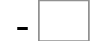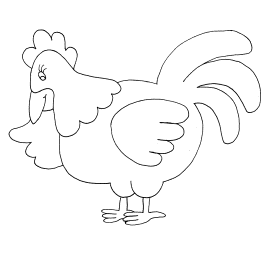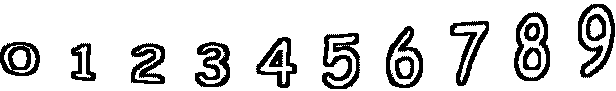9= 4   8= 26= 2   5= 0   1= 0   1= 1 6= 5   8= 7   5= 5   8= 5